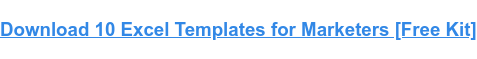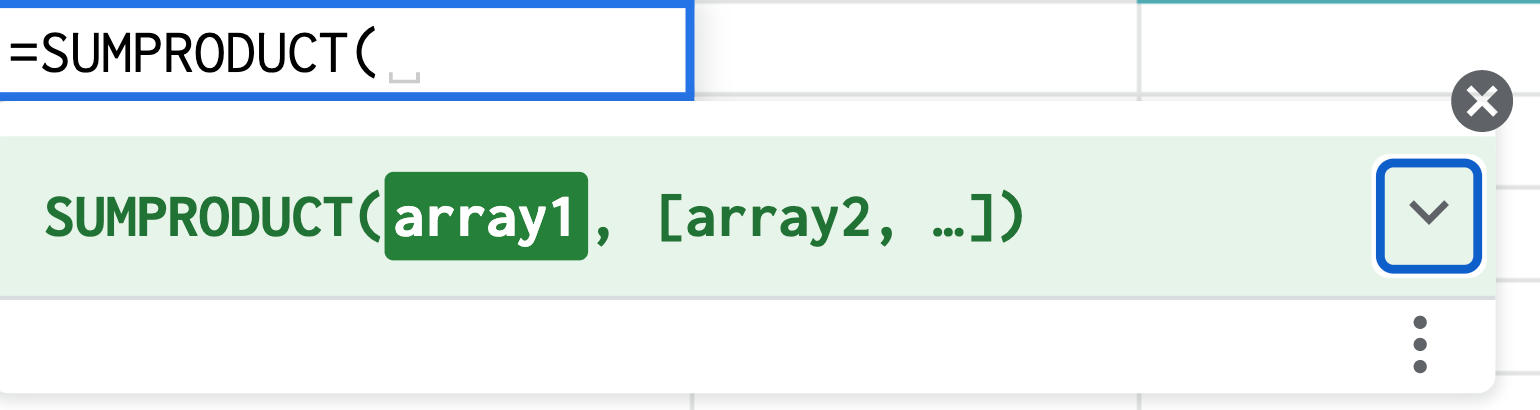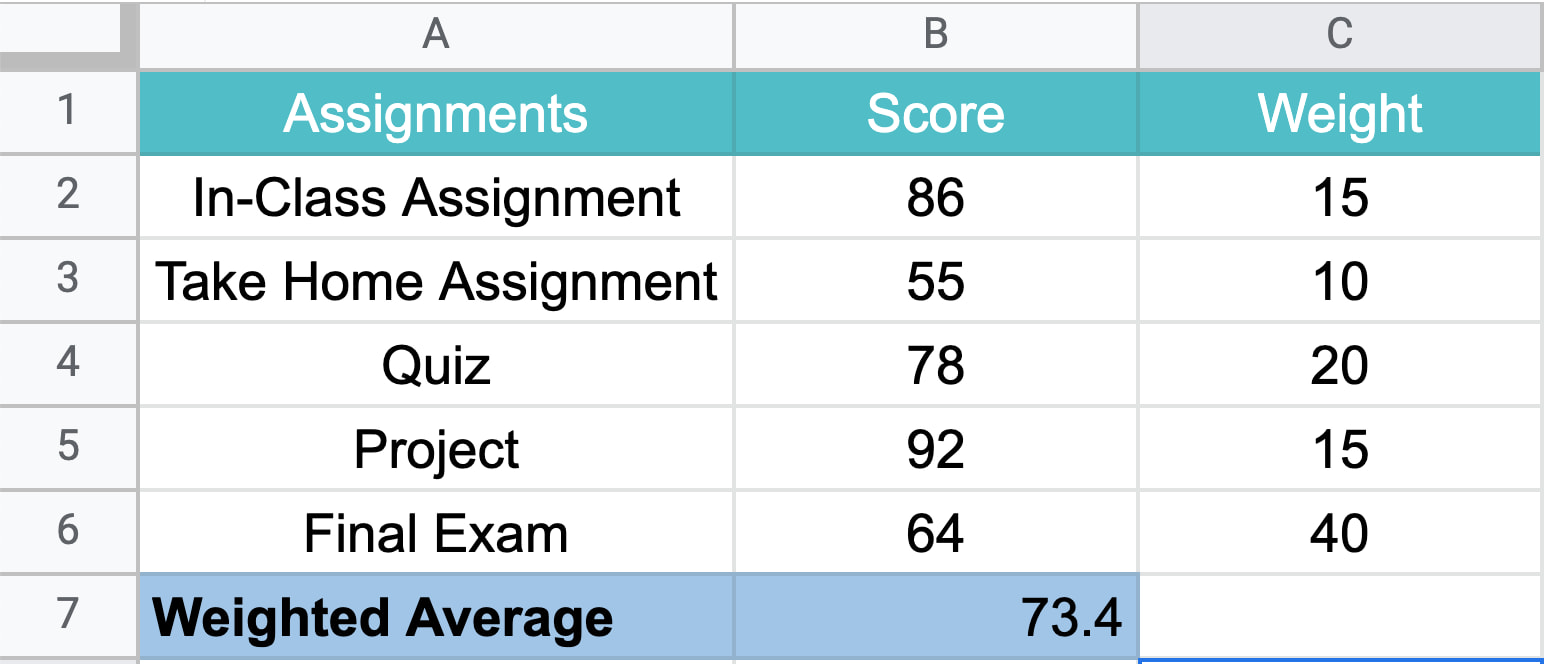# How to Use the Weighted Average Formula in Excel

When averaging a set of values, you usually work with values ​​that have the same weight and importance.

But what if some values ​​weigh more than others? This is where the weighted average formula comes in.In this article, we will explain how to use this formula in Excel, as well as provide some examples.

## How to calculate the weighted average in ExcelTo calculate the weighted average in Excel, you must use the SUMAPRODUCT and SUM functions using the following formula:

= SUMPRODUCT (X: X, X: X) / SUM (X: X)

This formula works by multiplying each value by its weight and combining the values. Then divide the SUMPRODUCT but the sum of the weights of your weighted average.

Are you still confused? Let’s review the steps in the next section.

## Use SUMPRODUCT to calculate the weighted average in Excel

### 3. Click Enter to get your results.## How to Find Weighted Moving Averages in Excel

A weighted moving average is a technique used to keep the average time period the same as you add new data or give more weight to certain time periods. This can help you identify trends and patterns more easily.

For example, if you have the number of views your website has had in the last five days, you can easily determine the average number of views in a five-day period.

Now, for example, next week, I ask for an average of five days, you would use the data from last five days, not the original five days of the previous week.

As such, you still rely on the same time period, but update the data to generate the moving average.

For a weighted moving average, give more weight to certain time periods than others. You can tell that day 5 weighs 60% and the remaining percentages decrease with the day.

Therefore, you will need to calculate this formula manually.

WMA = [value 1 x (weight)] + [value 2 x (weight)] + [value 3 x (weight)] + [value 4 x (weight)]

Once you understand this, using the weighted average formula is easy. All it takes is a little practice.